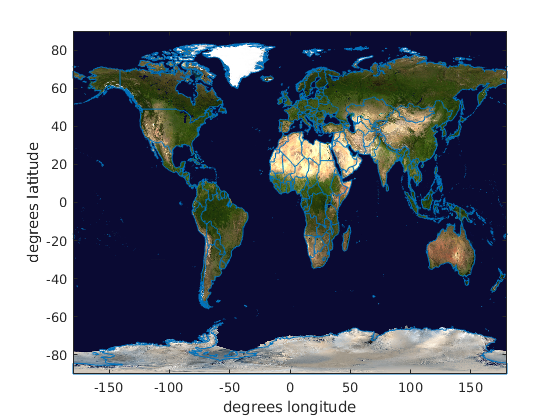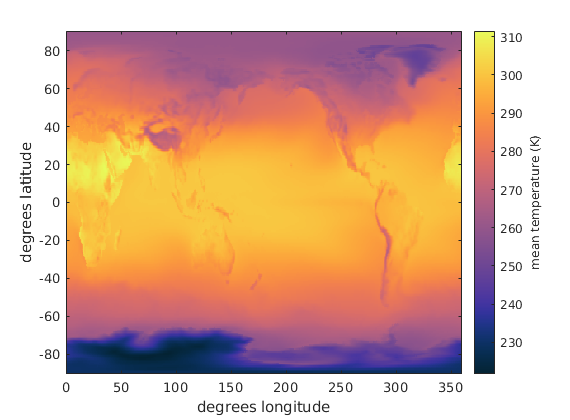# Mapmaking in Matlab

Back to Climate Data Tools Contents

There are many ways to make maps in Matlab. This tutorial reviews some of the strengths and weaknesses of each method.

## Contents

In the examples below, we'll map surface pressure, temperature, and wind data from the example ERA_Interim_2017.nc using several different methods. Start by loading the data. For more on loading NetCDF data, check out this tutorial.

```filename = 'ERA_Interim_2017.nc';

```

## Unprojected coordinates

The simplest and most computationally efficient way of plotting gridded Earth datasets is in unprojected coordinates. That means we make no attempt to account for the fact that the grid cells in a lat,lon grid are smaller near the poles than at the equator.

Here is a simple unprojected map of the Earth made using the earthimage function, with political boundaries overlaid using the borders function:

```earthimage % creates background image
hold on    % allows plotting more objects
borders    % draws political boundaries

xlabel 'degrees longitude'
ylabel 'degrees latitude'
```In the map above, you see that longitude is equivalent to the x axis and latitude is effectively the y axis. That means we can use regular plotting functions, treating longitude just like x, and latitude just like y. For example, to plot a line from New York (40N,74W) to Sydney (34S,151E), just use the standard plot function like this:

```% A line from New York to Sydney:
plot([-74 151],[40 -34],'ro-','linewidth',2)
```## imagesc and imagescn

Using standard plotting functions when making maps is convenient, simple, somewhat intuitive, and makes sense in some contexts. It's also nice beause no special toolboxes are required. And perhaps the biggest advantage is speed. When gridded datasets get large, special mapping functions that warp the grid into projected coordinates can be slow and take up a lot of memory. So if you ever run into problems with plotting speed, your first line of defense should be imagesc. Or better yet, use the CDT function imagescn, which automatically flips the y axis to the correct orientation and makes NaN grid cells transparent.

Here's the mean surface temperature plotted with imagescn, with a colormap set by cmocean. We'll also use quiversc to show the mean wind vectors:

```figure
imagescn(lon,lat,mean(T,3)')
cmocean thermal
cb = colorbar;
ylabel(cb,'mean temperature (K)')
xlabel 'degrees longitude'
ylabel 'degrees latitude'
```## pcolor

In the map above, we had to transpose (rotate by 90 degrees using the ' operator) the mean temperature grid to orient it correctly. The reason for that is explained in the NetCDF Tutorial.

If you don't want to mess with rotating grids, you can use pcolor instead of imagesc or imagescn, but you'll have to turn the lat,lon arrays into grids first, like this:

```% Turn lat,lon arrays into grids:
[Lat,Lon] = meshgrid(lat,lon);

figure
pcolor(Lon,Lat,mean(T,3))
cmocean thermal
xlabel 'degrees longitude'
ylabel 'degrees latitude'
```Note that pcolor always needs to be followed by shading flat or shading interp to get rid of the grid of black lines that usually makes the entire map look like a solid black rectangle. There are also some performance issues with pcolor which are discussed in the imagescn documentation, but it's still often a useful function.

Since we now have Lat,Lon grids that we generated from the lat,lon arrays, we can now use other functions too, like quiversc to draw the mean global wind fields:

```hold on % allows plotting more stuff on top of the pcolor plot
quiversc(Lon,Lat,mean(u10,3),mean(v10,3))
borders('countries','center',180)
```Above, we used the borders function to plot political boundaries, but we had to specify a center longitude of 180 degrees because this grid goes from 0 to 360 rather than from -180 to 180.

If you want to recenter the underlying data so the Prime Meridian (or any arbitrary longtidue) is at the center of the grid, simply use the recenter function:

```[Lat,Lon,T,u10,v10,sp] = recenter(Lat,Lon,T,u10,v10,sp);

figure
pcolor(Lon,Lat,mean(T,3))
cmocean thermal
borders
xlabel 'degrees longitude'
ylabel 'degrees latitude'
```In the map above, you see that now that we've recentered the gridded data, 0 degrees longitude is in the middle of the map and we didn't have to specify a center longitude when calling the borders function.

## Topography

Sometimes it's nice to show data in the context of surface tography. To get elevations at each point on the Lat,Lon grid, use the topo_interp funtion:

```Z = topo_interp(Lat,Lon);
```

Now use surf just the same as we used pcolor above, but with Z for the surface elevtions, and sp for surface pressure:

```figure
surf(Lon,Lat,Z,mean(sp,3))
cmocean dense
borders
xlabel 'degrees longitude'
ylabel 'degrees latitude'
caxis([950 1028]*100) % sets color axis limits
```The 3D view isn't very insightful, so change to 2D view with view(2) and use shadem to add some relief shading:

```view(2)             % sets 2D viewing angle
axis image          % removes whitespace and sets 1:1 aspect ratio
```## Projected coordinates

Although there are some advantages to using unprojected coordinates, there are also some drawbacks. Namely, unprojected coordinates are not very pretty, and they tend to exaggerate space near the poles while minimizing the relative size of grid cells near the equator. That can give a false impression of where the important physics are happening. Unprojected coordinates are also introduce unwanted distortion in regional maps, especially as you get closer to the poles.

If you want to make a map in projected coordinates you have two options:

2. * M_Map:* A free alternative to Matlab's Mapping Toolbox is M_Map by Rich Pawlowicz. M_Map is well written, well documented, well maintained, and it is compatible with Octave. It can produce publication-quality maps, and its only real drawback is it's one more thing to download.

## Mapping Toolbox

To make a map with Matlab's Mapping Toolbox, begin by initializing a map projection, and then use plotting functions in a manner quite similar to the unprojected examples above. The only differences here, are instead of using plot(lon,lat), pcolor(lon,lat,z), etc., you'll use plotm(lat,lon), pcolorm(lat,lon,z)., etc. In other words, put the letter m at the end of the function name you'd use in unprojected coordinates, and make sure the order is lat,lon rather than lon,lat.

If your work focuses on a specific region, specify that region by name when you call worldmap or give the geographic extents of the map you want to initialize, and the worldmap function will automatically figure out a decent projection. For this example, plot the whole world:

```figure
worldmap('world') % initializes a map of the world
```Now follow the same steps as we did with the pcolor map above, but use pcolorm and <bordersm_documentation.html bordersm:

```pcolorm(Lat,Lon,mean(T,3))
cmocean thermal
bordersm
xlabel 'degrees longitude'
ylabel 'degrees latitude'
cb = colorbar;
ylabel(cb,'temperature (K)')
```Unfortunately, we can't use quiverm to display the wind vectors, as we did in the unprojected example, because quiverm has some strange scaling issues. (Note: quiverm also mixes up the u and v vector components!) Thus, we've already run into one of the many little idiosyncracies that makes Matlab's Mapping Toolbox somewhat difficult to work with.

## M_Map

The M_Map website has many examples of how to create maps in Matlab using their program. It's a great package that's worth checking out.

## Globes

CDT offers a suite of functions for plotting on a globe. Globes may not be the best choice for displaying some types of data, because half the world's data is always on the other side of the globe, and a majority of what you can see is distorted around the perimeter of view. Globes are also computationally expensive because everything needs to be plotted in 3D space. However, desite the drawbacks of globes, then can still be very useful for exploring relationships between datasets, as you can manually rotate the globe at your leisure and develop an intuition for the true global spatial relationships between datasets. Globes are also good for making dynamic, eye-catching images that can engage a viewer.

The fastest way to make a globe is with the globeimage function. Here it is in action:

```figure
globeimage
```If you're following along, you can click on the globe and move it around to whatever viewing angle you wish. You can also begin to use the globe plotting functions to add more layers of data to the globe. The globe plotting functions mostly follow the form globeplot, globepcolor, globeborders, etc. For a full list of globe plotting functions see the CDT Contents page.

With the globeimage initialized, you can now add other data such as surface pressure contours, like this:

```globecontour(Lat,Lon,mean(sp,3),(950:10:1030)*100)
```Now add mean winds with globequiver like this:

```globequiver(Lat,Lon,mean(u10,3),mean(v10,3),'density',75)
```## Acknowledgements

This tutorial was written by Chad A. Greene, December 2018.